APPENDICES APPENDIX 5/2

Derivation of Uniform Series Payment Formulae

UNIFORM SERIES PAYMENT FORMULAE

If we invest an equal amount  R at the end of each period for a duration of  n periods at an interest rate  i per interest period, these investments will yield a Final Sum  S at the end of the  n periods.

The following diagrammatic illustration will facilitate your understanding of the inter-relationship between these Uniform Series Payment Formulae parameters.

Uniform (i.e. equal in amount) Series of Payments (R)

i = interest rate per
interest period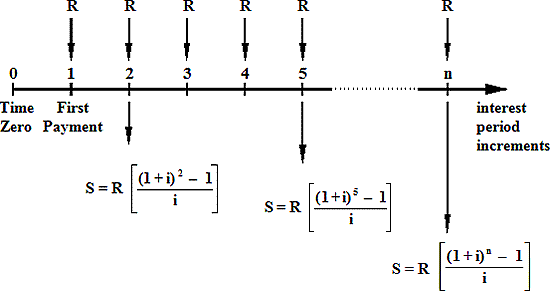InterestPeriod Sum at start ofInterest Period Sum at End of Interest Periods, S(including the End of Period investment, R) 1 Nil R 2 R R(1 + i ) + R 3 R(1 + i ) + R R(1 + i )2 + R(1 + i ) + R 4 R(1 + i )2 + R(1 + i ) + R R(1 + i )3 + R(1 + i )2 + R(1 + i ) + R 5 R(1 + i )3 + R(1 + i )2 + R(1 + i ) + R R(1 + i )4 + R(1 + i )3 + R(1 + i )2 + R(1 + i ) + R n R(1 + i )n-2 + R(1 + i )n-3 +......+ R(1 + i ) + R R(1 + i )n-1 + R(1 + i )n-2 +........... + R(1 + i ) + R

It is can be seen from the table above that Sn (the Sum at the End of n interest periods) is a Geometric Series.

It can also be seen from the development of the expressions for the 'Sum at the End of Interest Periods, S, that our Uniform Series Payment Formulae could be derived using Mathematical Induction.

Here, we will develop the formulae from the Geometric Series.

The general form for a Geometric Series is:

Sn = [a + ar + ar2 + ar3 + .......... + arn-2 + arn-1]

For the general Geometric Series formula:

Sn = a + ar + ar2 + ar3 + ar4+ .......... + arn-2 + arn-1

rSn = ar + ar2 + ar3 + ar4 + ar5+ .......... + arn-1 + arn

Sn - rSn = a - arn

Sn (1 - r )  =  a (1 - rn )

Sn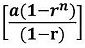where  a is the first term, and  r is the common ratio.

In the current situation:     Sn =  R(1 + i )n-1 + R(1 + i )n-2 +........... + R(1 + i ) + R

i.e. Sn =  R[(1 + i )n-1 + (1 + i )n-2 +........... + (1 + i ) + 1]

We can therefore apply the mathematical proofs from the general Geometric Series formula to our current situation.

The current situation can therefore be assessed as follows:

Sn =  RIn this Geometric Series:  a = (1 + i )n-1 and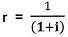Substituting for  a and  r in the Geometric Series formula yields:

Sn = R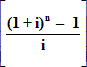Hence the following formulae :-

Formula (F3)S=RFormula (F4)R=S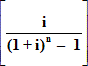But from Formula (F1) (see Appendix 5/1) we know that the Final Sum S at the end of n interest periods can also be realised by investing a Present Sum  P at interest rate  i per interest period over the  n periods.

i.e.   S=P ( 1 + i )n

Substituting this expression for S in Formula (F4) gives :

R=P (1 + i)nHence the following formulae:-

Formula (F5)R=P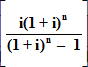Formula (F6)P=R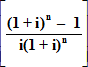So if we invest a Present Sum P for n interest periods at interest rate i per interest period this would mature to a Final Sum S at the end of n periods.

i.e.S = P( 1 + i )n

However instead of allowing our investment to mature to the Final Sum S at the end of the n periods we may withdraw equal amounts R at the end of each period over the n periods.

i.e.   R=PWhen you borrow money the lending institution is effectively investing a sum of money in you, the borrower. Therefore if we borrow a Present Sum P, as in an Annuity (Repayment) Mortgage, over n interest periods at an interest rate i per interest period, we repay the loan by repaying equal amounts R at the end of each of the interest periods.

Therefore formula (F5) is the formula used for computing repayments on an Annuity / Repayment Mortgage.

Illustration of Logarithmic Solution using Example 5.3

If we borrow a Present Sum (P) of £7,581.57 at an interest rate of 10% p.a. and we repay this loan by repaying £2,000 (R) at the end of each year, then at the end of what time period will our loan be fully repaid?

R=P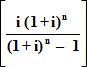£2,000      = £7,581.57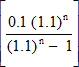0.2637976 =2.637976 [(1.1)n - 1]  = (1.1)n

2.637976 (1.1)n - 2.637976  =  (1.1)n

(1.1)n (2.637976 - 1.1)  = 2.637976

(1.1)n = 1.610509556

n log (1.1)   =   log (1.610509556)

n  =  5 years